# ISEE Upper Level Quantitative : How to subtract exponential variables

## Example Questions

### Example Question #1 : How To Subtract Exponential Variables

Simplify the expression: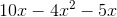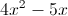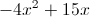The expression cannot be simplified further.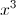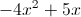Explanation:

Group, then collect like terms.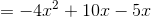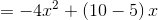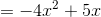### Example Question #2 : How To Subtract Exponential Variables

Assume neithernoris zero.

Which is the greater quantity?

(a)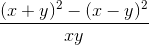(b)It is impossible to tell from the information given.

(a) and (b) are equal.

(b) is greater.

(a) is greater.

(a) and (b) are equal.

Explanation:

Simplify the expression in (a):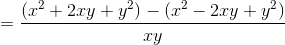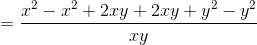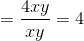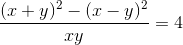regardless of the values of the variables, and (a) and (b) are equal.

### Example Question #3 : How To Subtract Exponential Variablesis a positive number.is a negative number.

Which is the greater quantity?

(a)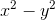(b)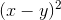(a) and (b) are equal.

It is impossible to tell from the information given.

(b) is greater.

(a) is greater.

(b) is greater.

Explanation: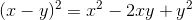is a positive number andis a negative number, so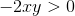and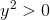. Therefore,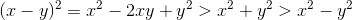.

(b) is always greater.